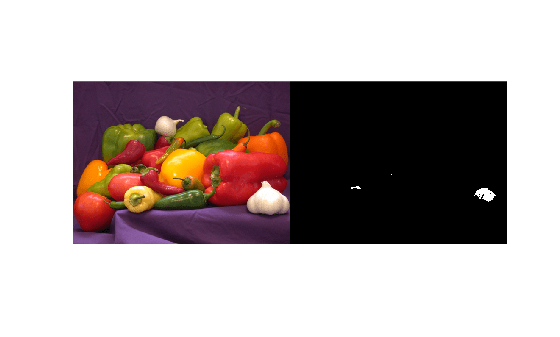## Syntax

``data = read(ds)``
``````[data,info] = read(ds)``````

## Description

example

````data = read(ds)` returns data from a datastore. Subsequent calls to the `read` function continue reading from the endpoint of the previous call.```
``````[data,info] = read(ds)``` also returns information about the extracted data in `info`, including metadata.```

## Examples

collapse all

Create a datastore from the sample file, `airlinesmall.csv`, which contains tabular data.

`ds = tabularTextDatastore('airlinesmall.csv','TreatAsMissing','NA','MissingValue',0);`

Modify the `SelectedVariableNames` property to specify the variables of interest.

`ds.SelectedVariableNames = {'DepTime','ArrTime','ActualElapsedTime'};`

While there is data available to be read from the datastore, read one block of data at a time and analyze the data. In this example, sum the actual elapsed time.

```sumElapsedTime = 0; while hasdata(ds) T = read(ds); sumElapsedTime = sumElapsedTime + sum(T.ActualElapsedTime); end```

View the sum of the actual elapsed time.

`sumElapsedTime`
```sumElapsedTime = 14531797 ```

Create a datastore from the sample file, `mapredout.mat`, which is the output file of the `mapreduce` function.

`ds = datastore('mapredout.mat');`

Read a subset of data in the datastore.

`T = read(ds)`
```T=1×2 table Key Value ______ _________ {'AA'} {} ```

Change the number of key-value pairs to read at a time, by changing the `ReadSize` property of the datastore.

`ds.ReadSize = 5;`

Read the next five key-value pairs in the datastore.

`T = read(ds)`
```T=5×2 table Key Value ______ _________ {'AS'} {[ 2910]} {'CO'} {[ 8138]} {'DL'} {} {'EA'} {[ 920]} {'HP'} {[ 3660]} ```

Create a datastore that maintains parity between the pair of images of the underlying datastores. For instance, create two separate image datastores, and then create a combined datastore that reads corresponding images from the two image datastores.

Create an image datastore `imds1` representing a collection of three images.

`imds1 = imageDatastore({'peppers.png','street1.jpg','street2.jpg'}); `

Create a second datastore `imds2` containing a mask of the bright regions of the three images. To create this datastore, first transform the images of `imds1` to grayscale. Then convert each image to a binary mask by performing thresholding. In this example, the thresholding operation maps pixels with a value above the threshold (`250`) to white and all other pixels to black.

`imds2 = transform(imds1,@(x) rgb2gray(x)>250);`

Create a combined datastore from `imds1` and `imds2`.

`imdsCombined = combine(imds1,imds2);`

Read the first subset of data from the combined datastore. The output is a 1-by-2 cell array. The two columns represent the first subset of data read from the two underlying datastores `imds1` and `imds2`, respectively.

`dataOut = read(imdsCombined)`
```dataOut=1×2 cell array {384x512x3 uint8} {384x512 logical} ```

Display the read data from the combined datastore as a pair of tiled images.

```tile = imtile(dataOut); imshow(tile)```Read from the combined datastore again. This call to the `read` function continues reading from the endpoint of the previous call.

`dataOut = read(imdsCombined)`
```dataOut=1×2 cell array {480x640x3 uint8} {480x640 logical} ```

```tile = imtile(dataOut); imshow(tile)```## Input Arguments

collapse all

Input datastore. You can use these datastores as input to the `read` method.

## Output Arguments

collapse all

Output data, returned as a table or an array depending on the type of `ds`.

Type of DatastoreData type of `data`Description
`TabularTextDatastore` and `SpreadsheetDatastore`TableThe `SelectedVariableNames` property determines the table variables.
`ImageDatastore`Integer array

The dimensions of the integer array depend on the type of image:

• For grayscale images, `data` is m-by-n.

• For truecolor images, `data` is m-by-n-by-3.

• For CMYK Tiff images, `data` is m-by-n-by-4.

If the `ReadSize` property is greater than 1, then `data` is a cell array of image data corresponding to each image. The `read` function supports all image types supported by the `imread` function. For more information on the supported image types, see `imread`.

`KeyValueDatastore`TableThe table variable names are `Key` and `Value`.
`FileDatastore`VariesThe output is the same as the output returned by the custom read function, specified by the `'ReadFcn'` value.
`TransformedDatastore`VariesThe output is the same as the output of the transformation function `@fcn` specified in the `transform` method used to create the `TransformedDatastore`.
`CombinedDatastore`Varies

Contains the horizontal concatenation of the output of read from the corresponding underlying datastores.

Information about read data, returned as a structure array or a cell array of structure arrays.

• For MATLAB datastores and `TransformedDatastore`, `info` is a structure array that has fields with information about the datastore.

• For `CombinedDatastore`, `info` is a cell array of structure arrays. Each element of the cell array contains a structure with the relevant fields of the corresponding underlying datastore.

Information in the structure array depends on the type of the input datastore. The structure array can contain the following fields.

Field NameDatastore TypesDescription
`Filename``ImageDatastore`,`SpreadsheetDatastore`, `TabularTextDatastore`, `FileDatastore`, `KeyValueDatastore`, and `TallDatastore``Filename` is a fully resolved path containing the path string, name of the file, and file extension. For `ImageDatastore` objects whose `ReadSize` property is greater than 1, `Filename` is a cell array of file names corresponding to each image.
`FileSize`

Total file size, in bytes.

For `ImageDatastore` objects whose `ReadSize` property is greater than 1, `FileSize` is a vector of file sizes corresponding to each image.

For MAT-files, the value of `FileSize` depends on the type of the datastore.

• `KeyValueDatastore` and `TallDatastore` — The `FileSize` field contains the total number of key-value pairs in the file.

• `FileDatastore` — The `FileSize` field contains the total file size in bytes.

`FileType``KeyValueDatastore` only

The type of file from which data is read, either `'mat'` for MAT-files or `'seq'` for sequence files.

`Label``ImageDatastore` only

Image label name. If the `ReadSize` property is greater than 1, then `Label` is a vector of label names corresponding to each image. If the `Labels` property is empty, then `Label` is an empty cell array.

`NumCharactersRead``TabularTextDatastore` only

`NumDataRows``SpreadsheetDatastore` only

Vector containing number of rows read from each sheet.

`Offset``KeyValueDatastore` and `TabularTextDatastore` only

Starting position of the read operation, in bytes. For MAT-files, `Offset` is the index of the first key and value read.

`SheetNames``SpreadsheetDatastore` only

`SheetNumbers``SpreadsheetDatastore` only